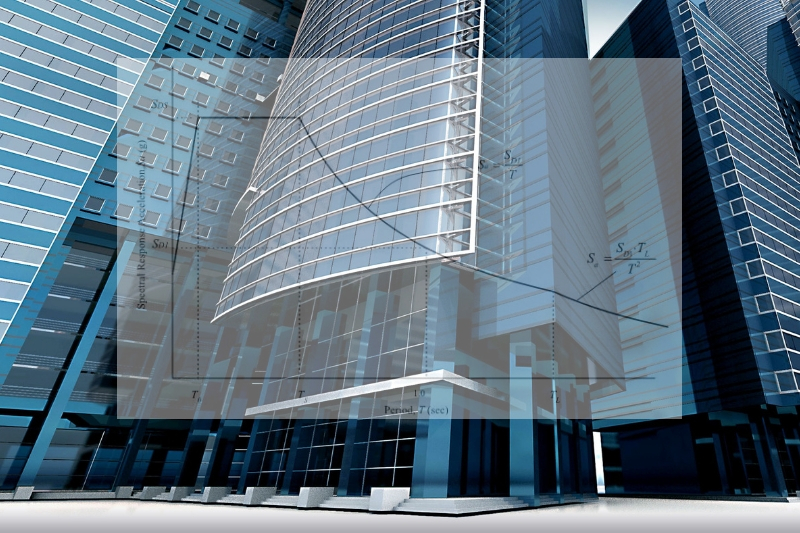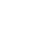Scaling of Base Shears | The Structural World# Scaling of Base Shear Results of Static and Dynamic AnalysisBase shear is an estimate of the maximum expected lateral force that will occur at the base of the structure due to the seismic ground motion. During the analysis, the codes required for the use of the equivalent static force procedure and a dynamic lateral force procedure.  Hence, the base shear obtains or calculated from the dynamic analysis should be reduced to a certain percentage of the base shear results that is determined from the static force procedure. Now, the big question is why we need to reduce the elastic response parameters calculated by dynamic analysis and how we are going to do that? To answer those questions let us first differentiate static analysis to that of dynamic analysis.

## Static and Dynamic Analysis

We all know that Static and Dynamic Analysis is usually done by a computer program. To differentiate on where it can be used, Static Analysis is used basically for regular structures under 240 feet (72 meters) and for those irregular structures that are 65 feet (19.5 meters) or less. On the other hand, Dynamic Analysis is required for regular structures higher than 240 feet and 65 feet tall for irregular structures.

## Why do we need to reduce the Elastic Response parameter and How?

A Scale Factor or scaling of the results is used in the analysis to reduce the elastic response parameters. This is usually done to normalize the forces and to distribute it properly while the code level forces are being maintained. A different approach according to the code we use, e.g. UBC-97, ASCE 7 or IBC has its own interpretation of how it is done. Let us refer to the succeeding explanation in order to further understand this concept. Two different approaches using UBC-97 and ASCE-7 will be discussed accordingly.

## 1. Static and Dynamic Lateral Force Procedure using UBC-97

#### Static Force Procedure

The base shear result of the Static Force Procedure can be determined using UBC-97 code. Refer to the previous article Seismic Analysis: UBC-97 Provisions for the determination of Linear Static Seismic Lateral Force.

#### Dynamic Lateral Force Procedure

UBC-97 1631.5.4 requires that if the base shear determined by a dynamic analysis is less than that of the static lateral force procedure, it must be scaled to equal that determined by the equivalent static force procedure.  Similarly, if the base shear obtained from the dynamic analysis is greater than that specified by the static lateral force procedure, it may be scaled down.

In Summary:

VDYNAMIC < VSTATIC → VDYNAMIC  to scaled to equal

VDYNAMIC > VSTATIC → VDYNAMIC  to scaled down

#### How to Apply the above Scaling Principle in Analysis Model?

Scaling of the analysis results can be further understood by an example. Since this article is composed of scaling principles, let’s leave it to that. Nevertheless, a separate article was published regarding the step by step procedures on actual scaling using an ETABS model. Refer to this link for an example.

## 2. Static and Dynamic Lateral Force Procedure using ASCE 7-10

#### Static Force Procedure

The base shear result of the Static Force Procedure can be also determined using ASCE-7 code. Compared to UBC-97, ASCE-7 has a similarity obtaining the base shear except that the determination of static seismic lateral forces differs only according to the seismic parameters. You can refer to ASCE-7 for further information.

#### Dynamic Lateral Force Procedure

According to ASCE 7-10 section 12.9.2 Modal Response Parameters summarizes that the value for each force for each mode of response shall be computed using the properties of each mode and the response spectra shall be multiplied by g/R*I (section 12.9.2) where g is the gravitational acceleration of 9.81m/s2, R is the numerical coefficient representative of the inherent over strength and global ductility capacity of lateral force-resisting frame and I is the importance factor.

In Summary:

Initial scale to use:  g/R*I

The above initial scaling factor should be confirmed in accordance with section 12.9.4.1, Scaling of Forces states that where the combined response for the modal base shear (Vt) is less than 85 % of the calculated base shear (V) using the lateral force procedure, the forces shall be multiplied by 0.85(V/Vt) where V is the equivalent lateral force procedure base shear as per ASCE 7-10 section 12.8 and Vt is the base shear required from the modal combinations.

Hence:

If Vt  > 0.85V → No further scaling required

If  Vt < 0.85V →  (g/R*I) x 0.85 (V/Vt)

#### How to Apply the above Scaling Principle in Analysis Model?

Scaling of the analysis results can be further understood by an example. Since this article is composed of scaling principles, let’s leave it to that. Nevertheless, a separate article will be published soon on the step by step procedures on actual scaling using an ETABS model. So stay tuned for that.

Tell us your thoughts. Leave your comment below and subscribe to our newsletter for the upcoming updates.

12,500 total views,  4 views today

Copyright secured by Digiprove © 2018-2019 The Structural World
Advertisements

## 17 Comments

1.What if the dynamic base shear is larger than the static and why?

1.Rico Gomez

If the code being used is ASCE 7-10 and base shear of the dynamic analysis using the initial scale factor is bigger than the equivalent lateral force procedure then no further scaling is required.

2.thestructuralworld (Post author)

If the dynamic base shear is larger, it should be reduced to a certain percentage of the base shear results. Further details on why we are scaling, please read the article carefully.

3.prabhu r

Sir how about in Indian code

4.thestructuralworld (Post author)

For Indian Code, Response spectrum method of analysis shall be performed using the design spectrum specified in Clause 6.4.2 or by a site-specific design, spectrum mentioned in Clause 6.4.6 of IS 1893.

1.Manohar

hi please state Indian code requirements for dynamic analysis and the checks that are to be performed for the tall structure.

5.Mangesh

Sir, can u tell me how to give torsion in structure? Like 5%, 10%, or 20%?

1.thestructuralworld (Post author)

You can refer to Table M of UBC 97 for the requirements.

6.MANGESH NAGDEVE

Why we are using base shear why not torsion? Base shear is maximum, I know but in some part torsion also occurred please explain?

1.thestructuralworld (Post author)

Torsional irregularity is a separate check. The designer should review the structural arrangement of the elements to ensure the irregularity requirements of UBC97 Clause 1629.9.3, and tables’ 16-L or 16-M are satisfied.

7.Davide

Honestly I cannot find in the above explanation why “base shear scaling” is required.
Only few codes require base scaling.
e.g. the New Zealand and Australia codes require it. An explanation I found regarding those codes refers back to the first seismic code issued, where RSA was a new approach and therefore not fully “tested”.
The ESA is a very simplified analysis that considers only the first mode of vibration and applies to regular structures only.
I therefore consider very conservative increasing a base shear to match a base shear that is based on assumptions that do not apply to a more complex and irregular structure where multiple modes of vibration contribute to the overall dynamic behaviour, especially if the first mode of vibration is torsional and engages only 20 or 30% of the mass.

8.thestructuralworld (Post author)

As mentioned in the above article, UBC-97 section 1631.5.4 and ASCE-7 section 12.9.4.1 Elastic Response Parameters may be reduced thus, base shear scaling is required to normalize the forces and to distribute it properly and It can be applied to regular or irregular structures as specified in the code.

9.Hardik Mulay

What if our fifth mode is torsional of building of G+30?

10.Adrian Jimenez

1. What is the use of having an initial value of g/R if you are going to scale it up to 85% of the static base shear anyways? Can you have an arbitrary number instead?

2. Is the scaled dynamic base shear be the one to use for checking seismic drifts?

1.thestructuralworld (Post author)

1. This is to convert the spectrum into mm/sec2. Some designers tends to use the gravitational acceleration of 9810 mm/sec2. But as far as the code is concern, g/R should be use.
2. Yes definitely!

11.Nabil Otrie

I carefully read the article , I couldn’t find a logical reason to do so ( scaling down) , only this word “ Similarly….”
As well known that the spec base shear is minimum, so how to reduce it!?
Please clarify more about the reason with reference if possible

12.thestructuralworld (Post author)

UBC-97 1631.5.4 requires that if the base shear determined by a dynamic analysis is less than that of the static lateral force procedure, it must be scaled to equal that determined by the equivalent static force procedure. If the base shear obtained from the dynamic analysis is greater than that specified by the static lateral force procedure, it may be scaled down. Refer to this link for example: https://www.thestructuralworld.com/2019/05/24/2-methods-on-how-to-scale-base-shear-in-etabs/